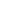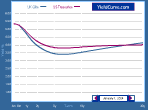Home   What's New   Market Research   Presentations### EURO-FUTURE STRIP HEDGE

Abukar M AliThis worksheet is an educational tool designed to demonstrate the workings of the Bloomberg TED page. A primary use of this page is to calculate the hedge required for a holding of a cash bond, when this hedge is constructed using exchange-traded 90-day interest-rate futures contracts. The user can use the worksheet to calculate an approximation of the futures strip required to hedge a long or short position in a cash bond. For illustration, we show the TED page when it has been used to calculate the strip hedge for a \$100m long position in the Treasury 3 7/8% 2013. PLEASE NOTE: this example is for a bond whose maturity date extends beyond the maturity of the longest-dated futures contract. If the bond has a maturity date that does not extend beyond a certain contract, then these remaining should not be considered.
##### Description
Nominaliamountm The nominal value of the bond position being hedged
Spread The difference between the bond yield-to-maturity and the implied futures rate
Contract This is the specific contract used in the strip. The designation follows the exchange-traded custom, with H, M, U and Z used to designate contracts expiring in March, June, September and December each year
Days The term to maturity of the specified futures contract
Price The price of the futures contract
Rate The implied 90-day Libor rate on maturity implied by the price of the futures contract
Di The discount factor derived from the futures contract, given by 1 + (EDi-TED) * days_i/360 for spread adjusted hedging
Face value The nominal amount of the bond position
Present value The present value of the face value, given by Payment * (di*di2*di3* ...*din)^-1
Nr of contracts The number of contracts required in the futures strip to hedge the bond holding

Home   What's New   Market Research   Presentations
RATE yield curve model   Excel Spreadsheets© YieldCurve.comDisclaimerweb design